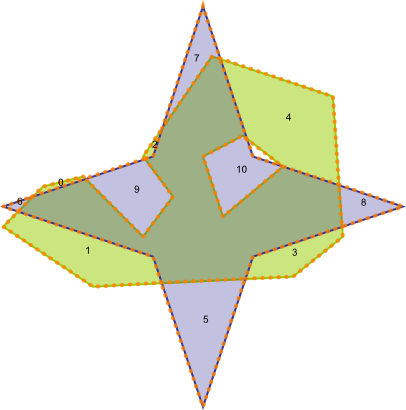#Boost C++ Libraries

...one of the most highly regarded and expertly designed C++ library projects in the world.

This is the documentation for an old version of Boost. Click here to view this page for the latest version.

#### sym_difference

Calculate the symmetric difference of two geometries.

###### Description

The free function symmetric difference calculates the spatial set theoretic symmetric difference (XOR) of two geometries.

###### Synopsis

```template<typename Geometry1, typename Geometry2, typename Collection>
void sym_difference(Geometry1 const & geometry1, Geometry2 const & geometry2, Collection & output_collection)```

###### Parameters

Type

Concept

Name

Description

Geometry1 const &

Any type fulfilling a Geometry Concept

geometry1

A model of the specified concept

Geometry2 const &

Any type fulfilling a Geometry Concept

geometry2

A model of the specified concept

Collection &

output collection, either a multi-geometry, or a std::vector<Geometry> / std::deque<Geometry> etc

output_collection

the output collection

###### Header

Either

`#include <boost/geometry.hpp>`

Or

`#include <boost/geometry/algorithms/sym_difference.hpp>`

###### Conformance

The function sym_difference implements function SymDifference from the OGC Simple Feature Specification.

###### Behavior

Case

Behavior

areal (e.g. polygon)

All combinations of: box, ring, polygon, multi_polygon

linear (e.g. linestring) / areal (e.g. polygon)

A combinations of a (multi) linestring with a (multi) polygon results in a collection of linestrings

linear (e.g. linestring)

All combinations of: linestring, multi_linestring; results in a collection of linestrings

pointlike (e.g. point)

All combinations of: point, multi_point; results in a collection of points

Other geometries

Not yet supported in this version

Spherical

Not yet supported in this version

Three dimensional

Not yet supported in this version

NoteCheck the Polygon Concept for the rules that polygon input for this algorithm should fulfill
###### Example

Shows how to calculate the symmetric difference (XOR) of two polygons

```#include <iostream>

#include <boost/geometry.hpp>
#include <boost/geometry/geometries/polygon.hpp>
#include <boost/geometry/geometries/point_xy.hpp>
#include <boost/geometry/geometries/multi_polygon.hpp>

#include <boost/foreach.hpp>

int main()
{
typedef boost::geometry::model::polygon<boost::geometry::model::d2::point_xy<double> > polygon;

polygon green, blue;

boost::geometry::read_wkt(
"POLYGON((2 1.3,2.4 1.7,2.8 1.8,3.4 1.2,3.7 1.6,3.4 2,4.1 3,5.3 2.6,5.4 1.2,4.9 0.8,2.9 0.7,2 1.3)"
"(4.0 2.0, 4.2 1.4, 4.8 1.9, 4.4 2.2, 4.0 2.0))", green);

boost::geometry::read_wkt(
"POLYGON((4.0 -0.5 , 3.5 1.0 , 2.0 1.5 , 3.5 2.0 , 4.0 3.5 , 4.5 2.0 , 6.0 1.5 , 4.5 1.0 , 4.0 -0.5))", blue);

boost::geometry::model::multi_polygon<polygon> multi;

boost::geometry::sym_difference(green, blue, multi);

std::cout
<< "green XOR blue:" << std::endl
<< "total: " << boost::geometry::area(multi) << std::endl;
int i = 0;
BOOST_FOREACH(polygon const& p, multi)
{
std::cout << i++ << ": " << boost::geometry::area(p) << std::endl;
}

return 0;
}
```

Output:

```green XOR blue:
total: 3.1459
0: 0.02375
1: 0.542951
2: 0.0149697
3: 0.226855
4: 0.839424
5: 0.525154
6: 0.015
7: 0.181136
8: 0.128798
9: 0.340083
10: 0.307778```
###### See also
 Copyright © 2009-2014 Barend Gehrels, Bruno Lalande, Mateusz Loskot, Adam Wulkiewicz, Oracle and/or its affiliates Distributed under the Boost Software License, Version 1.0. (See accompanying file LICENSE_1_0.txt or copy at http://www.boost.org/LICENSE_1_0.txt)